American Journal of Theoretical and Applied Statistics
Volume 4, Issue 3, May 2015, Pages: 78-84

Robust Linear Regression Using L1-Penalized MM-Estimation for High Dimensional Data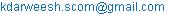(K. Darwish)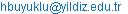(A. H. Buyuklu)

Kamal Darwish, Ali Hakan Buyuklu. Robust Linear Regression Using L1-Penalized MM-Estimation for High Dimensional Data. American Journal of Theoretical and Applied Statistics. Vol. 4, No. 3, 2015, pp. 78-84. doi: 10.11648/j.ajtas.20150403.12

Abstract: Large datasets, where the number of predictors p is larger than the sample sizes n, have become very popular in recent years. These datasets pose great challenges for building a linear good prediction model. In addition, when dataset contains a fraction of outliers and other contaminations, linear regression becomes a difficult problem. Therefore, we need methods that are sparse and robust at the same time. In this paper, we implemented the approach of MM estimation and proposed L1-Penalized MM-estimation (MM-Lasso). Our proposed estimator combining sparse LTS sparse estimator to penalized M-estimators to get sparse model estimation with high breakdown value and good prediction. We implemented MM-Lasso by using C programming language. Simulation study demonstrates the favorable prediction performance of MM-Lasso.

Keywords: MM Estimate, Sparse Model, LTS Estimate, Robust Regression

1. Introduction

In modern real-world applications, high-dimensional datasets, where the total number of variables p is much larger than sample size n, but the number of important variables is typically smaller than n, are not uncommon. Examples are gene expression microarray data and functional Magnetic Resonance Imaging (fMRI) data. A typical goal in sparse high-dimensional model fitting is to ensure high prediction accuracy and discovering relevant predictive variables. However, ill-conditioned design matrix in high-dimensional model makes statistical estimation is fundamentally different from the estimation problems in the classical settings.

Regularized or penalized estimations have been widely used to overcome the computational problems with high- dimensional data and to improve prediction accuracy. A penalty parameter can be added to the objective function on the regression coefficients to tradeoff between variance and bias as ridge estimation . A popular approach is the least absolute shrinkage and selection operator (Lasso)  that uses the L1 penalty.

Consider the linear regression problem in the matrix form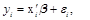(1)

where y is an n-vector of random responses, X an n × p design matrix, β a p-vector of parameters, and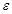an n-vector of iid random errors have zero expected value. With a penalty parameter λ, the Lasso estimate ofis(2)

where the loss function L is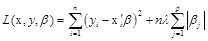(3)

As with ridge regression (Lasso) shrinks the coefficients, however. L1-penalty forces some of the coefficient estimates to be exactly equal to zero if the tuning parameter λ is sufficiently large, i.e., to produce sparse model estimates that are highly interpretable. Hence, much like best subset selection, the Lasso performs variable selection. It can effectively select important explanatory variables and estimate regression parameters simultaneously. In contrast to classical L0 penalized variable selection methods, AIC, BIC, CP and so on, the Lasso is computationally feasible for high-dimensional data. A fast algorithm for computing the Lasso is available through the framework of least angle regression (LARS) . The satisfactory finite-sample performance of Lasso under normal errors has been demonstrated numerically in , and its statistical properties have been studied (e.g [4,5]).

The main drawback of the Lasso is that it is not robust to outliers. The breakdown point of the Lasso is 1/n  i.e., even a single outlier can severely distort the Lasso estimate completely.

To produce more robust estimator than Lasso, the least absolute deviations (LAD) regression is combined with Lasso regression to produce an estimator called LAD-lasso ,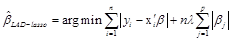(4)

All estimators mentioned until now, are a special case of a more general estimator, the penalized M-estimator ,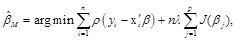(5)

with loss function ρ : R R and penalty function J : R R. Using convex loss functions make these estimators non robust with respect to leverage points and result in a breakdown point of 1/n .

A robust version of ridge regression was proposed by , using L2 penalized MM-estimators. Even though the resulting estimates are not sparse, prediction accuracy is improved by shrinking the coefficients, and the computational issues with high-dimensional robust estimators are overcome due to the regularization.

Ref  proposed a robust estimator with respect to leverage points, called RLARS. RLARS is a robust version of the stepwise algorithm LARS which is computationally very efficient but sensitive to outliers. A main drawback of the RLARS algorithm is the lack of a natural definition, since it is not optimizing a clearly defined objective function.

A popular robust estimator is the least trimmed squares (LTS) estimator . Although its simple definition and fast computation make it interesting for practical application, it cannot be computed for high-dimensional data (p > n). Combining the Lasso estimator with the LTS estimator, developed the sparse LTS-estimator ,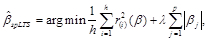(6)

where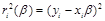denotes the squared residuals and r2(1) (β) ≤ . .. ≤ r2(n) (β) their order statistics. Here λ ≥ 0 is a penalty term and h ≤ n the size of the subsample that is deemed to consist of non-outlying observations. This estimator can be applied to high-dimensional data with good prediction performance and high robustness. It also has a high breakdown point .

However, sparse LTS can be applied to high-dimensional data, it should be noted that its efficiency is an issue. In this paper, we attempt to combine reweighting sparse LTS estimator with penalized M-estimators (Tukey’s biweight functions with L1-penalty). We employ the approach of MM estimation which was first proposed in ; using sparse LTS as an initial estimator for computing L1-penalized M-estimator yields L1- penalized MM-estimator.

The rest of the paper is organized as follows. In Section 2, we illustrate the proposed algorithm to combine high breakdown value estimation and efficient estimation. Section 3 presents the results of a simulation study that compares the performance of the estimated models by the root mean squared prediction error (RMSPE). In addition concerning sparsity, the estimated models are evaluated by the false positive rate (FPR) and the false negative rate (FNR). The results indicate that, L1-Penalized MM-estimation yields a model that achieves excellent prediction accuracy with a sparse representation of the predictors in the model. Finally, Section 4 concludes.

2. L1-Penalized MM-Estimation

To combine high breakdown value estimation with efficient estimation under the normal model, the approach of MM estimation is employed. The L1-Penalized MM-estimation (henceforth MM-Lasso) can be constructed by a three-stage procedure. In the first stage, we compute an initial consistent estimator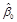with high breakdown point(BDP) but possibly low normal efficiency. In the second stage, we compute a robust M-scale estimator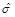of the residuals based on the initial estimate. In the third stage, we compute an L1-Penalized M estimator with fixed scale; starting the iterations from; and using a loss function that ensures the desired efficiency. Here, efficiency will be loosely defined as similarity with the classical lasso estimator at the normal model.

Let ρ0(r) = ρBI(r/k0), ρ(r) = ρBI(r/k1), and assume that each of the ρ-functions is bounded even in the sense of . The scale M estimator (an M-scale for short)satisfies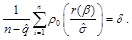(7)

Where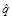is the number of non-zero estimated parameters in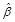which depends on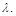To obtain consistency when the errors are normal, the constant d satisfies d = EΦ [ρ (Z)], with Φ the standard normal distribution. Note that if ρ(t) = t2 and d = 1 then= s the residual standard deviation . The MM-Lasso is defined with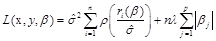(8)

where the factor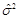before the summation is employed to make the estimator coincide with the classical one when ρ(t) = t2. Let ρ satisfy ρ ≤ ρ0, from  it is easy to show ifsatisfies L(x, y,) ≤ L(x, y,), then’s BDP is not less than that of. The value of k0 should be chosen in order to attain high breakdown point of the MM-Lasso. The choice of k1 will to determine asymptotic efficiency of the estimate without affecting its breakdown point. In order to let ρ ≤ ρ0 , we must have k1 ≥ k0; the larger the k1 is, the higher efficiency the MM-Lasso can attain at the normal distribution.

2.1. IRLS Algorithm

The penalty function in equation (2) is convex but it is a non-differentiable function, hence it is difficult to obtain analytic form solution of equation (2). Here we can obtain an approximate closed form solution as in :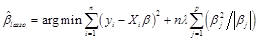(9)

where the L1 Penalization had a similar form to an L2 norm with a weight |βj|. Iteratively re-weighted least squares (IRLS) algorithm for the Lasso estimate in equation (9) can be obtained by computing the ridge regression iteratively as:(10)

where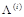is the generalized inverse (pseudo-inverse) of matrix= diag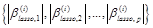and i = 0,1... is the iteration number. Similarly, an iteratively re-weighted least squares (IRLS) algorithm for the MM-Lasso estimate. Define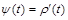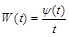(11)

Let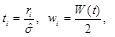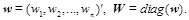(12)

by differentiating of (5) with respect to β and setting the derivative to zero, one gets,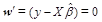(13)

and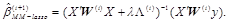(14)

Since for the chosen ρ, W(t) is a decreasing function of |t|, observations with larger residuals will receive lower weight w. The iteration will stop until a maximum number is reached or the difference between two successive iteration steps is small enough.

The following is the procedure to obtain the estimator1)         A high breakdown estimator is used to find an initial estimate(in this paper we choose sparse LTS estimator in ). Using this estimate the residuals, ri () =, are computed, for 1≤ i ≤ n.

2)         Using these residuals from the robust fit, an M-estimate of scalewith high BDP is computed from (6).

3)         At each iteration withremains fixed throughout, calculate residuals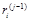and associated weight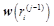according to the weight function.

4)         Solve the following for iteratively re-weighted least squares (IRLS) equation,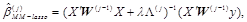Steps 3) and 4) are repeated until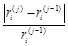becomes less than tolerance.

2.2. Weight Functions and Choosing the Constants

Several types of weight functions are proposed for IRLS algorithm in literature. Each set of functions given includes tuning constants, which allow for the shape of the function to be slightly altered. Beaton and Tukey  proposed the IRLS algorithm with Tukey’s bisquare function that enables to remove the influence of extreme outliers completely from the estimation.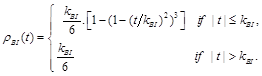(15)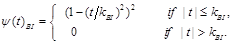(16)

where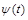=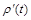is called a redescending function, and the value kBI for bisquare function is a tuning constant. In particular, The value k0 =2.937 such that the asymptotically consistent scale estimatehas the breakdown value of 25%, while the value k1 = 3.44 yields 0.85 asymptotic efficiency at the normal model when λ = 0 .

However, the scale estimaterequires a correction for high dimensional data. According to , there are two problems appear when fitting a standard MM estimator to data with a high ratio p/n:

(1)       The scale based on the residuals from the initial regression estimator underestimates the true error scale.

(2)       Even if the scale is correctly estimated, the actual efficiency of the MM estimator can be much lower than the nominal one. For this reason,is corrected using (9) in  aswith k1 = 1.29, k2 = - 6.02.

2.3. Choosing the Penalty Parameter

We propose to select λ by the estimated prediction error of MM-Lasso for different values of λ via cross-validation. We can use the k-fold cross validation process, which requires recomputing the estimate k times. For k = n ("leave-one-out") we can use an approximation to avoid recomputing.

Call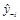the fit of yi computed without using the i-th observation; i.e., y-i =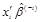, where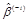is the MM-Lasso estimate computed without observation i. Then a first-order Taylor approximation of the estimator yields the approximate prediction errors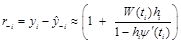(16)

with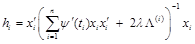where,and ti are defined in (11)-(12), xi is the i-th row of X defined in (13) andis the generalized inverse (pseudo-inverse) defined in (10). Given the prediction errors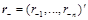, we compute a robust mean squared error (MSE) as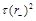, where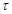is a "-scale" with tuning constant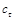= 5 , and choose the λ minimizing this MSE.

3. Simulation Study

To investigate the behaviour of our robust estimator, a simulation study for comparing the performance of various sparse estimators are performed in R (R Development Core Team, 2011) with package simFrame, which is a general framework for simulation studies in statistics. As in  we make a comparison with the lasso, the LAD-Lasso, robust least angle regression (RLARS) and Sparse LTS with reweighted step. Sparse LTS is evaluated for the subset size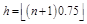to guarantee a breakdown point of 25%. All computations are carried out in R version 3.1.2 (R Development Core Team, 2011) using the packages robustHD  for sparse LTS and RLARS, quantreg  for the LAD-Lasso and lars  for the Lasso. We implemented MM-Lasso by using C programming language.

For every generated sample, an optimal value of the shrinkage parameter λ is selected. The penalty parameters for MM-Lasso are chosen using k-fold cross validation process as described in subsection 2.4, and the other methods are optimized via BIC as described in .

3.1. Sampling Schemes

In this study we take the three configurations from  to represent low, moderate and high dimensional data. Firstly in the case of n > p, we create a linear model. From k = 6 latent independent standard normal variables, L1 , L2 , ... , Lk and an independent normal error variable e with standard deviation σ, the response variable y is constructed as

y = L1 + L2 + · · · + Lk + σε,      (16)

The value of σ is chosen so that the signal to noise ratio is equal to 3. A set of p = 50 candidate predictors is then constructed as follows. Let e1, ..., ed be independent standard normal variables and let

Xi = Li + τei, i = 1, ..., k

Xk+1 = L1 + δek+1

Xk+2 = L1 + δek+2

Xk+3 = L2 + δek+3

Xk+4 = L2 + δek+4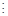X3k-1 = Lk + δe3k-1

X3k = Lk + δe3k

and Xi = ei , i = 3k + 1, ..., p

The constants δ = 5 and τ = 0.3 are chosen so that corr(X1, Xk+1) = corr(X1, Xk+2) = corr(X2, Xk+3) = · · · = corr(Xk, X3k) = 0.5. Note that covariates X1, ..., Xk are "low noise" perturbations of the latent variables and constitute our "target covariates". Variables X3k+1, ..., Xd are independent noise covariates and variables Xk+1, ..., X3. The number of observations is set to n = 150.

The case of moderate high-dimensional data is represented by the second configuration. We generate n = 100 observations from a p-dimensional normal distribution N(0, Σ), with p = 250. The covariance matrix Σ = (Σ ij) 1≤i,j≤p is given by Σij = 0.5|i−j|, creating correlated predictor variables. Using the coefficient vector β = (βj) 1≤ j≤ p with β1 = β7 = 1.5, β2 = 0.5, β4 = β11 = 1, and βj = 0 for j {1, . . . , p}\{1, 2, 4, 7, 11}, the response variable is generated according to the regression model (1), where the error terms follow a normal distribution with σ = 0.5.

Finally, the third configuration covers the case of high dimensional data with n = 100 observations and p = 500 variables. The first 250 predictor variables are generated from a multivariate normal distribution N(0, Σ) with Σij = 0.6|i−j|. Furthermore, the remaining 250 covariates are standard normal variables. Then the response variable is generated according to (1), where the coefficient vector β = (βj) 1≤ j≤ p is given by βj = 1 for 1 ≤ j ≤ 10 and βj = 0 for 11 ≤ j ≤ p, and the error terms follow a standard normal distribution.

To allow for a fraction of outliers we considered the following sampling distributions, listed in increasing order of difficulty

1. No contamination.

2. Vertical outliers: 10% of the error terms in the regression model follow a normal N(20, σ) instead of a N(0, σ). 3. Leverage points: Same as in 2, but the 10% contaminated observations contain high-leverage values by drawing the predictor variables from independent N(50,1) distributions.

4. The outliers form a dense cluster: Keeping the contamination level at 10%, outliers in the predictor variables are drawn from independent N(10, 0.01) distributions. Let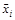denote such a leverage point. Then the values of the response variable of the contaminated observations are generated by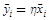with γ = (−1/p) 1≤j≤p. The parameter η controls the magnitude of the leverage effect and is varied from 1 to 25 in five equidistant steps.

3.2. Simulation Results

In this subsection, the results for the different data scheme are presented and discussed. The performance of the estimated models are compared by the root mean squared prediction error (RMSPE). For this purpose, we generate n additional observations from the respective sampling schemes (without outliers) as test data, and this in each simulation run. The RMSPE of the oracle estimator, which uses the true coefficient values β, is computed as a standard for the evaluated methods. In addition considering sparsity, the estimated models are evaluated by the false positive rate (FPR) and the false negative rate (FNR). Both FPR and FNR should be as small as possible for a sparse estimator. RMSPE, FPR and FNR, averaged over 100 simulation runs, are reported for every method.

3.2.1. The First Sampling Scheme

The simulation results for the first data are represented in table 1. It can be seen that when there is no contamination in the data LAD-Lasso, RLARS and Lasso have excellent performance in RMSPE and FPR, while sparse LTS and MM-Lasso have a good prediction, but they have larger FPR than other methods. In addition, MM-Lasso improves the estimates of sparse LTS, which is reflected in the lower values for RMSPE and FPR. On the other hand, there are no false negatives in all of these methods.

In the case of vertical outliers, the higher values of RMSPE and FPR show that Lasso is non-robust estimator.

All of methods are still have excellent performance in RMSPE but sparse LTS and MM-Lasso have considerable values of FPR. As showed in Table 1 RMSPE and FPR of MM-Lasso are 1.1765, 0.237 while sparse LTS have RMSPE and FPR equals 1.2378, 0.293 respectively. Ultimately, MM-Lasso has a significant improvement over Sparse LTS. In the third scenario, when we introduce leverage points in addition to vertical outliers, RLARS, MM-Lasso, and sparse LTS have a good performance. However, the RMSPE and FPR of RLARS increased (1.1210 to 1.2236, and 0.029 to 0.126, respectively) also the FPR of sparse LTS (0.293 to 0.319) and MM-Lasso (0.237 to 0.250) slightly increase. MM-Lasso still increases the performance of sparse LTS in RMSPE and FPR (1.1792 and 0.250 respectively). LAD-lasso has large RMSPE and suffers from false positives, while Lasso has large RMSPE and FNR. This suggests that the leverage points have a bad leverage effect.

Figure 1 refers to the results for the fourth contamination setting. The RMSPE for the more robust methods is plotted as a function of the parameter η. RLARS has a considerably higher RMSPE than MM-Lasso and sparse LTS for lower values of η, but the RMSPE gradually decreases with increasing η. The RMSPE of sparse LTS in beginning slightly increased then decreased in the next steps. However, MM-Lasso has the lowest RMSPE; thus, their overall performance is the best.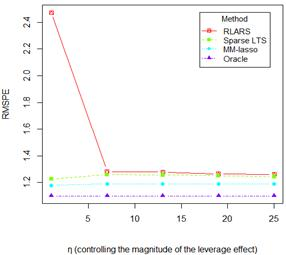Figure 1. Root mean squared prediction error (RMSPE) for the first simulation scheme, with n =150 and p = 50, and for the fourth contamination setting.

Table 1. Results for the first simulation scheme, with n = 150 and p = 50.

 Method No contamination Vertical outliers Leverage points RMSPE FPR FNR RMSPE FPR FNR RMSPE FPR FNR Lasso 1.1778 0.080 0.000 1.7376 0.225 0.088 2.5205 0.066 0.766 LAD-Lasso 1.1316 0.092 0.000 1.1640 0.161 0.000 1.9939 0.316 0.002 RLARS 1.1450 0.066 0.000 1.1210 0.029 0.000 1.2236 0.126 0.030 Sparse LTS 1.2623 0.265 0.000 1.2378 0.293 0.000 1.2345 0.319 0.000 MM-Lassoa 1.1705 0.213 0.000 1.1765 0.237 0.000 1.1792 0.250 0.000 Oracle 1.1073 1.1073 1.1073 a Proposed method.

3.2.2. The Second Sampling Scheme

Table 2 shows the simulation results for the second data configuration (the moderate high-dimensional data). In the case without contamination, MM-Lasso, and RLARS have the best performance. Also, the LAD-Lasso and Lasso have excellent prediction performance but a slightly higher FPR than the other methods, followed by sparse LTS. In the case of vertical outliers, RLARS still has excellent prediction performance despite some false negatives. We notice that RMSPE and FPR of MM-Lasso are 0.5766 and 0.034 respectively. While, for Sparse LTS are 0.6688, 0.039 respectively. Hence, MM-Lasso achieves good sparse prediction without false negative. Drastically, Lasso is non-robust against vertical outliers.

In the scenario with additional leverage points, it can be concluded that sparse LTS has RMSPE equal 0.6691 and FPR equal 0.039 also MM-Lasso has RMSPE equal 0.5738 and FPR equal 0.035. It means that there is stability in these methods. For RLARS, there is small increase in the RMSPE, FPR and FNR. On the other hand, LAD-Lasso already has a considerably large RMSPE, and again a slightly higher FPR than the other methods. Furthermore, the Lasso is still highly influenced by the outliers, which is reflected in a very high FNR and poor prediction performance. Briefly, compared to other methods MM-Lasso is deemed a best performance.

Figure 2 clarifies the results for the fourth contamination setting. As in first scheme, we plotted the RMSPE for the more robust methods. The RMSPE of RLARS is gradually decreasing. The RMSPE of MM-Lasso and sparse LTS have constant low values. MM-Lasso clearly performs best for all values of η.

Table 2. Results for the second simulation scheme, with n = 100 and p =250.

 Method No contamination Vertical outliers Leverage points RMSPE FPR FNR RMSPE FPR FNR RMSPE FPR FNR Lasso 0.5848 0.105 0.000 2.3551 0.185 0.092 2.6857 0.013 0.632 LAD-Lasso 0.6020 0.067 0.000 0.7446 0.011 0.000 1.8398 0.096 0.112 RLARS 0.5506 0.016 0.000 0.6092 0.015 0.055 0.7901 0.072 0.098 Sparse LTS 0.7195 0.028 0.000 0.6688 0.039 0.000 0.6691 0.039 0.000 MM-Lassoa 0.5526 0.022 0.000 0.5766 0.034 0.000 0.5738 0.035 0.000 Oracle 0.4998 0.4998 0.4998 a Proposed method.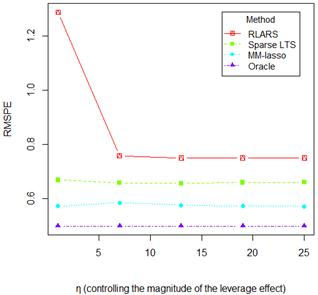Figure 2. Root mean squared prediction error (RMSPE) for the second simulation scheme, with n =100 and p = 250, and for the fourth contamination setting.

3.2.3. The Third Sampling Scheme

Table 3 presents the simulation results for the high dimensional data configuration. When the data is free from contamination, the sparse LTS is characterized as the lowest efficiency due to have larger values of RMSPE than other methods. In the other hand, MM-Lasso and RLARS have considerably better performance in this case. Lasso and LAD-Lasso have a good behavior. With vertical outliers, the RMSPE for the Lasso increases extremely due to a high FNR, while LAD-Lasso still has good prediction performance. In addition, RLARS has a larger FNR, resulting in a slightly lower RMSPE. When leverage points are introduced, MM-Lasso exhibits the lowest RMSPE and sparse LTS keep its excellent behavior.

Figure 3 shows the results for the fourth contamination setting. It can be seen that RMSPE of RLARS is higher in the beginning, and then decreases continuously in the remaining steps. MM-Lasso and Sparse LTS have low and constant values for RMSPE but MM-Lasso is close to Oracle.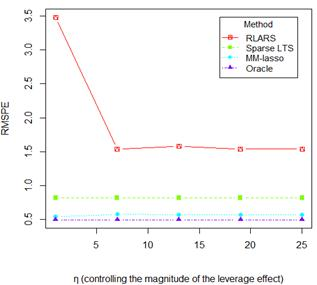Figure 3. Root mean squared prediction error (RMSPE) for the third simulation scheme, with n =100 and p =500, and for the fourth contamination setting.

3.3. Summary of the Simulation Results

This study shows that MM-Lasso has RMSPE values close to Oracle, and does not suffer from any false positives at all. Hence, MM-Lasso is the best overall performance. Sparse LTS generally have good prediction accuracy; however, MM-Lasso can improve this prediction. Although RLARS has good achievement, contamination data makes FNR values increased. These simulation results also enhance that the Lasso is not robust to outliers and LAD-Lasso is not robust against bad leverage points but is resistant to vertical outliers.

Table 3. Results for the third simulation scheme, with n =100 and p = 500.

 Method No contamination Vertical outliers Leverage points RMSPE FPR FNR RMSPE FPR FNR RMSPE FPR FNR Lasso 0.6114 0.073 0.000 3.3311 0.061 0.280 3.6073 0.054 0.350 LAD-Lasso 0.6493 0.023 0.000 0.8316 0.006 0.000 2.8667 0.074 0.132 RLARS 0.5767 0.009 0.000 0.8954 0.009 0.081 1.4805 0.052 0.112 Sparse LTS 0.9804 0.006 0.000 0.7912 0.005 0.000 0.7520 0.005 0.001 MM-Lassoa 0.5429 0.005 0.000 0.5626 0.004 0.000 0.5725 0.005 0.000 Oracle 0.4983 0.4983 0.4983 a Proposed method.

4. Conclusion

Sparse Least trimmed squares (Sparse LTS) is a robust, shrinkage and selection regression estimation with high breakdown value and good prediction estimation, However, it should be noted that efficiency is an issue with sparse LTS. Our proposed estimator MM-Lasso, an approach of MM estimation, used sparse LTS estimator as initial estimator to penalized M-estimators (Tukey’s biweight functions with L1-penalty). Our model, MM-Lasso can improve prediction estimation of sparse LTS and its overall performance is the best.

Acknowledgements

We would like to express our sincerely thanks to the Editor, and referees for their valuable and constructive comments that led to considerable improvement of the paper.

References

1. A. E. Hoerl and R. W. Kennard, "Ridge Regression: Biased Estimation for Nonorthogonal Problems," Technometrics, vol. 12, no. 1, pp. 55–67, 1970.
2. R. Tibshirani, "Regression shrinkage and selection via the lasso," J. Royal. Statist. Soc B., vol. 58, no. 1, pp. 267–288, 1996.
3. B. Efron, T. Hastie, and R.Tibshirani, "Least angle regression," The Annals of Statistics, vol. 32, pp, 407–499, 2004.
4. K. Knight and W. Fu,"Asymptotics for Lasso-Type Estimators,"The Annals of Statistics, vol. 28, pp. 1356–1378, 2000.
5. J. Fan and R. Li, "Variable Selection via Nonconcave Penalized Likelihoodand its Oracle Properties,"Journal of the American Statistical Association, vol. 96, no. 456, pp.1348–1360, 2001
6. A. Alfons, C. Croux, and S. Gelper, "Sparse least trimmed squares regression for analyzing high dimensional large data sets," The Annals of Applied Statistics, vol. 7, no. 1, pp. 226–248, 2013.
7. H.Wang, G. Li, and G. Jiang, "Robust regression shrinkage and consistent variable selection through the LAD-lasso,"Journal of Business & Economic Statistics, vol. 25, pp. 347-355, 2007.
8. G. Li, H. Peng, and L. Zhu,"Nonconcave penalized M-estimation with a diverging number of parameters," Statitica Sinica , vol. 21, no. 1, pp. 391–419, 2013.
9. R. A. Maronna, "Robust ridge regression for high-dimensional data," Technometrics, vol. 53, pp. 44–53, 2011.
10. J. A. Khan, Aelst, S. Van. and R. H. Zamar, "Robust linear model selection based on least angle regression," Journal of the Statistical Association, vol. 102, pp. 1289–1299, 2007.
11. P. Rousseeuw and A. Leroy, Robust regression and outlier detection. John Wiley & Sons, 1987.
12. V. J.Yohai, "High Breakdown-point and High Efficiency Estimates for Regression," The Annals of Statistics, vol. 15, pp. 642-65, 1987.
13. R. Maronna, D. Martin, and V. Yohai, Robust Statistics. John Wiley & Sons, Chichester. ISBN 978-0-470-01092-1, 2006.
14. A. E. Beaton, and J. W. Tukey,"The fitting of power series, meaning polynomials, illustrated on band-spectroscopic data,"Technometrics, vol. 16, pp. 147-185, 1974.
15. R. A. Maronna, and V. J. Yohai, "Correcting MM Estimates for Fat Data Sets," Computational Statistics & Data Analysis, vol. 54, pp. 3168-3173, 2010.
16. V. J. Yohai and R.H. Zamar, "High breakdown-point estimates of regression by means of the minimization of an efficient scale," Journal of the American Statistical Association, vol. 83, pp. 406–413, 1988.
17. A. Alfons, simFrame: Simulation framework. R package version 0.5, 2012b.
18. A. Alfons, robustHD: Robust methods for high-dimensional R pakage version 0.1.0, 2012a.
19. R. Koenker, quantreg: Quantile regression.R package version 4.67, 2011.
20. T. Hasti and B. Efron, lars: Least angle regression, lasso and forward stagewise. R package version 0.9-8, 2011.

 Contents 1. 2. 2.1. 2.2. 2.3. 3. 3.1. 3.2. 3.3. 4.
Article ToolsAbstractPDF(283K)